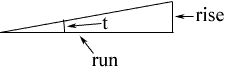Quandaries and Queries incline- % grade as to degree. ie 6% hwy grade= what degree of incline? Hi, A 6% grade means that in a straight section of the road the rise is 6% of the run. Thus in the diagram below the ratio of the rise to the run is 0.06.Thus the tangent of the angle t is 0.06 and t = tan-1(0.06) = 3.43 degrees. Penny Go to Math Central